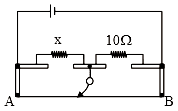A meter bridge is set up to determine unknown resistance x using a standard 10 $\Omega$ resistor. The galvanometer shows the null point when the tapping key is at a 52 cm mark. End corrections are 1 cm and 2 cm respectively for end A and B. Then the value of x is1.  10.2$\Omega$

2.  10.6$\Omega$

3.  10.8$\Omega$

3. 11.1$\Omega$